Explaining Dropout (Bayesian Deep Learning Part I)

Dropout is a now-ubiquitous regularization technique introduced by Hinton in 2012, and originally provided without any meaningful theoretical grounding. In a network with dropout, neurons are randomly turned on and off at training time, and the outputs are averaged at inference time. Intuitively, this creates a ensemble of classifiers, each of which focuses on slightly different features, thus preventing overfitting. This quora question explains it a bit more in depth in a few different ways.

While intuitively satisfying and generally accepted, this explanation of dropout lacks mathematical rigor. A Bayesian perspective, taken recently by Yarin Gal provides a mathematically grounded explanation of dropout, showing it is equivalent to minimizing the divergence between  true and approximate distributions over the network weights.

I’ve pulled the derivation that shows this for a single hidden layer network from his thesis, and added a bit of commentary based on my understanding of it.

Finding an Approximating Distribution

Withas our weights,our variational parameters, andandour training inputs and outputs, we find the best approximating distribution,, for our actual distribution,, by minimizing Kullback-Leibler divergence.   Or, in less words, we seek to minimize:Via the magic of Bayes’ theorem and logarithm rules, we can reach an alternate form of the loss: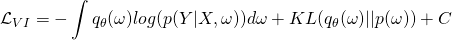We note that ourandare discrete, and reformat our log probability: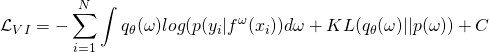Where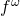represents the model output for a given input and weight parameters, and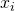andrepresent single inputs and outputs from our training set,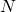is the number of training samples. As a constant,can (and will be) dropped from our optimization procedure.

Further, we note we can reformulate our final loss function using a subset of the training data:Where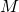is the size of our set. Theterm works as a normalizer, making sure our scale doesn’t change. This is important to show because dropout generates a sample population, not a predictable iteration through all datapoints.

Utilizing the pathwise derivative estimator, we arrive at a new loss function:whereis drawn from an as of yet undefined distribution.

Reformulating Dropout

We’ve reformulated our KL minimization, now we need to reformulate dropout to match. We start with a standard regularized loss function, whereandrepresent weights and biases with no dropout:In Consistent inference of probabilities in layered networks: Predictions and generalizations, it is shown that the squared error term is equivalent to: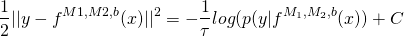If we designate a function, which corresponds to a dropout layer if the epsilon are a Bernoulli distribution, our fully formed loss function becomes:What this means

If you look closely, you can see how similarand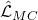are. Essentially, if we can select a prior,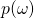, where the KL convergence is equal to our L2 penalty, they match to a scale.

Of course, due to the fact that it’s implemented via enabling and disabling neurons, we don’t ACTUALLY have to worry about this in implementation. But, mathematically, it turns out that by setting the prior to independent multivariate normals with appropriate variance, we can make this true.

Most importantly, though, this means that we can link mathematics related to the approximating distribution and KL estimation to our dropout, which becomes important when we talk about the idea of Bayesian Deep Learning (continued here if you want to read about regression, or here for classification).

This site uses Akismet to reduce spam. Learn how your comment data is processed.